# Levi-Civita connection exists and is unique

## Statement

Suppose$M$ is a differential manifold and$g$ is a Riemannian metric or a pseudo-Riemannian metric. Then, there is a unique linear connection on$M$ satisfying the following two conditions:

1. It is a metric connection.
2. It is a torsion-free linear connection.

This connection is called the Levi-Civita connection.

## Related facts

1. Corollary of Leibniz rule for Lie bracket: This states that:
•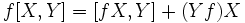$\! f[X,Y] = [fX,Y] + (Yf)X$.
•$\! f[X,Y] = [X,fY] - (Xf)Y$.

## Proof

### Formula for the Levi-Civita connection and proof of its uniqueness

Take three vector fields$X, Y, Z$. Now, consider the three equations obtained by cycling$X, Y, Z$ in the first condition. Solving this system of linear equations, we can express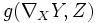$g(\nabla_XY,Z)$ in terms of$X,Y,Z$.

Explicitly: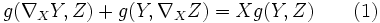$g(\nabla_XY,Z) + g(Y,\nabla_XZ) = Xg(Y,Z) \qquad (1)$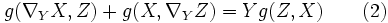$g(\nabla_YX,Z) + g(X,\nabla_YZ) = Yg(Z,X) \qquad (2)$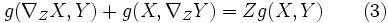$g(\nabla_ZX,Y) + g(X,\nabla_ZY) = Zg(X,Y) \qquad (3)$

Now let's choose to focus only on the clockwise cyclic expressions, that is, the three expressions$p = g(\nabla_XY,Z), q = g(\nabla_YZ,X), r = g(\nabla_ZX,Y)$.

Writing everything in terms of these three (we here make use of the torsion tensor vanishing):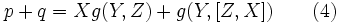$p + q = Xg(Y,Z) + g(Y,[Z,X]) \qquad (4)$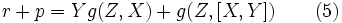$r + p = Yg(Z,X) + g(Z,[X,Y]) \qquad (5)$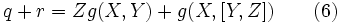$q + r = Zg(X,Y) + g(X,[Y,Z]) \qquad (6)$

Consider (4) + (5) - (6):$\! 2p = Xg(Y,Z) + Yg(Z,X) - Zg(X,Y) + g(Y,[Z,X]) + g(Z,[X,Y]) - g(X,[Y,Z])$.

This simplifies to: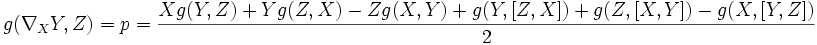$g(\nabla_XY,Z) = p = \frac{Xg(Y,Z) + Yg(Z,X) - Zg(X,Y) + g(Y,[Z,X]) + g(Z,[X,Y]) - g(X,[Y,Z])}{2}$.

Thus, the value$g(\nabla_XY,Z)$ is determined for all$Z$, as the expression on the right side. Moreover, since the Lie bracket of derivations is$\R$-bilinear and the metric$g$ is$\R$-bilinear, the right side is linear in$Z$. Thus, the function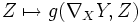$Z \mapsto g(\nabla_XY,Z)$ is linear. Finally, since$g$ is nondegenerate, there exists a unique vector field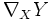$\nabla_XY$ such that$g(\nabla_XY,Z)$ is this function.

### The connection exists

We now argue that the connection$\nabla_XY$ defined in this way is a connection, i.e., it satisfies the conditions necessary for a connection. First, note that the expression is$\R$-linear in both$X$ and$Y$, so$\nabla_XY$ is$\R$-bilinear.

To check that the connection is$C^\infty$-linear in$X$, it suffices to show that the map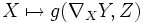$X \mapsto g(\nabla_XY,Z)$ is$C^\infty$-linear. We do this:$g(\nabla_{fX}(Y),Z) = \frac{fXg(Y,Z) + Y(fg(Z,X)) - Z(fg(X,Y)) + g(Y,[Z,fX]) + g(Z,[fX,Y]) - fg(X,[Y,Z])}{2}$.

We use the Leibniz rule:$g(\nabla_{fX}(Y),Z) = \frac{fXg(Y,Z) + (Yf)g(Z,X) + f(Yg(Z,X)) - (Zf)g(X,Y) - f(Zg(X,Y)) + g(Y,[Z,fX]) + g(Z,[fX,Y]) - fg(X,[Y,Z])}{2}$.

We now use fact (1):$g(\nabla_{fX}(Y),Z) = \frac{fXg(Y,Z) + (Yf)g(Z,X) + f(Yg(Z,X)) - (Zf)g(X,Y) - f(Zg(X,Y)) + fg(Y,[Z,X]) + (Zf)g(Y,X) + fg(Z,[X,Y]) - (Yf)g(Z,X) - fg(X,[Y,Z])}{2}$.

Cancelling and grouping terms gives: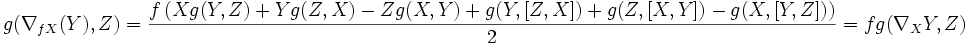$g(\nabla_{fX}(Y),Z) =\frac{f\left(Xg(Y,Z) + Yg(Z,X) - Zg(X,Y) + g(Y,[Z,X]) + g(Z,[X,Y]) - g(X,[Y,Z])\right)}{2} = fg(\nabla_XY,Z)$.

Next, we check the Leibniz rule property. In other words, we need to show that: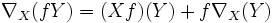$\nabla_X(fY) = (Xf)(Y) + f\nabla_X(Y)$.

To do this, it suffices to show that:$g(\nabla_X(fY),Z) = (Xf)g(Y,Z) + fg(\nabla_XY,Z)$.

We begin by expanding the left side: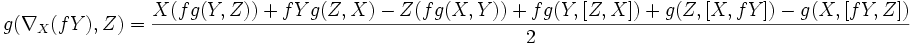$g(\nabla_X(fY),Z) = \frac{X(fg(Y,Z)) + fYg(Z,X) - Z(fg(X,Y)) + fg(Y,[Z,X]) + g(Z,[X,fY]) - g(X,[fY,Z])}{2}$

Applying the Leibniz rule: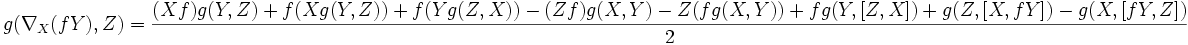$g(\nabla_X(fY),Z) = \frac{(Xf)g(Y,Z) + f(Xg(Y,Z)) + f(Yg(Z,X)) - (Zf)g(X,Y) - Z(fg(X,Y)) + fg(Y,[Z,X]) + g(Z,[X,fY]) - g(X,[fY,Z])}{2}$

Using fact (1) now yields: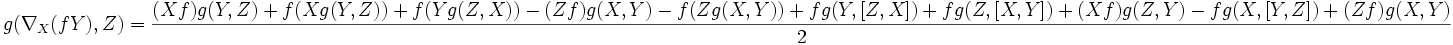$g(\nabla_X(fY),Z) = \frac{(Xf)g(Y,Z) + f(Xg(Y,Z)) + f(Yg(Z,X)) - (Zf)g(X,Y) - f(Zg(X,Y)) + fg(Y,[Z,X]) + fg(Z,[X,Y]) + (Xf)g(Z,Y) - fg(X,[Y,Z]) + (Zf)g(X,Y)}{2}$

Cancelling and grouping terms gives: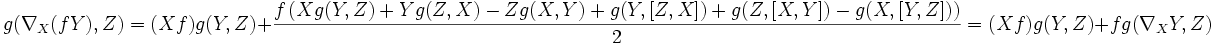$g(\nabla_X(fY),Z) = (Xf)g(Y,Z) + \frac{f\left(Xg(Y,Z) + Yg(Z,X) - Zg(X,Y) + g(Y,[Z,X]) + g(Z,[X,Y]) - g(X,[Y,Z])\right)}{2} = (Xf)g(Y,Z) + fg(\nabla_XY,Z)$.

This completes the proof.